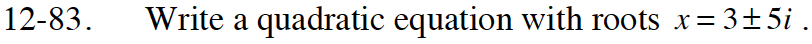Home > CCA2 > Chapter 12 > Lesson 12.1.4 > Problem12-83

12-83.

Write a quadratic equation with roots x = 3 ± 5i. Homework Help ✎If the roots are x = 3 ± 5i, then the factors must be (x − (3 + 5i)) and (x − (3 − 5i)).

Use the factors to write and simplify an equation.
(x − 3 + 5i)(x − 3 − 5i) = 0

x2 − 6x + 34 = 0